### Alcohol gel dispenser A4988 stepping motor + HC-SR04Code

```const int pingPin = 13;
int inPin = 12;

//////////////// step motor

#define dirPin 3
#define stepPin 4
#define stepsPerRevolution 800

void setup() {

Serial.begin(9600);
pinMode(stepPin, OUTPUT);
pinMode(dirPin, OUTPUT);
}

void loop()
{

long duration, cm;

pinMode(pingPin, OUTPUT);

digitalWrite(pingPin, LOW);
delayMicroseconds(2);
digitalWrite(pingPin, HIGH);
delayMicroseconds(5);
digitalWrite(pingPin, LOW);
pinMode(inPin, INPUT);
duration = pulseIn(inPin, HIGH);

cm = microsecondsToCentimeters(duration);

if (cm <= 15) {
motorFeed();
}
else {
motorStop();
}
Serial.print(cm);
Serial.print("cm");
Serial.println();
delay(100);

}

long microsecondsToCentimeters(long microseconds)
{
// The speed of sound is 340 m/s or 29 microseconds per centimeter.
// The ping travels out and back, so to find the distance of the
// object we take half of the distance travelled.
return microseconds / 29 / 2;
}

void motorFeed() {
// Set the spinning direction clockwise:
digitalWrite(dirPin, HIGH);
// Spin the stepper motor 1 revolution slowly:
for (int i = 0; i < stepsPerRevolution; i++) {
// These four lines result in 1 step:
digitalWrite(stepPin, HIGH);
delayMicroseconds(2000);
digitalWrite(stepPin, LOW);
delayMicroseconds(2000);
}
//delay(500);
// Set the spinning direction counterclockwise:
digitalWrite(dirPin, LOW);
// Spin the stepper motor 1 revolution quickly:
for (int i = 0; i < stepsPerRevolution; i++) {
// These four lines result in 1 step:
digitalWrite(stepPin, HIGH);
delayMicroseconds(2000);
digitalWrite(stepPin, LOW);
delayMicroseconds(2000);
}

}

void motorStop() {
// Set the spinning direction clockwise:
digitalWrite(dirPin, LOW);
// Spin the stepper motor 1 revolution slowly:
for (int i = 0; i < stepsPerRevolution; i++) {
// These four lines result in 1 step:
digitalWrite(stepPin, LOW);
delayMicroseconds(1000);
digitalWrite(stepPin, LOW);
delayMicroseconds(1000);
}

}

```

### Arduino MEGA 2560 Multiwii Drone: ESC assembly and how to use + Calibration

How to control motorStep

1. Low throttle
2. connect battery
3. Beep Beep
4. Di Di Di
5. Maximum Throttle

### Arduino MEGA 2560 Multiwii Drone : Add GPS + U-center change baud rate + update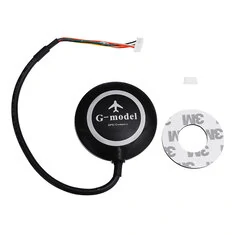connectGPS >>> FTDI

TX  >>> RX

RX >>> TX

https://www.u-blox.com/en/product/u-centerFile for update firmware

1. Unzip
2. open U-Center .### Arduino MEGA 2560 Multiwii Drone :Bluetooth Telemetry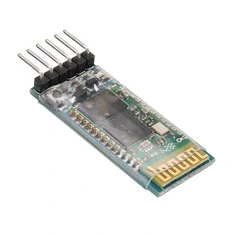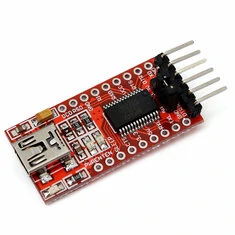ConnectFTDI                   Bluetooth

RX     >>>>        TX

TX     >>>>         RX

Open IDE Sketch the

Tool >  port > Com? (Select com port)

Type

AT enter for test OK mean good communicationChange Bluetooth Baud rate

Type

AT+BAUD8(change baud rate 115200)Connect Bluetooth  to Mega2560VDO

### Arduino MEGA 2560 Multiwii Drone :Test GPS

Test VDO

All component

1. Gyroscope + Accelerometer  (MPU6050)• UBlox GPS + Magnetometer (HMC5883)
• Magnetometer (HMC5883)G-Model GPS/Compass

Compass

Orange =  SCL

White = SDA

GPS

Green = TX

Yellow = RXCode program

How to configuration

### Arduino Drone : MEGA2560 Aititude Hold + GPS Hold EP1## Flight dynamics

Each rotor produces both a thrust and torque about its center of rotation, as well as a drag force opposite to the vehicle’s direction of flight. If all rotors are spinning at the same angular velocity, with rotors one and three rotating clockwise and rotors two and four counterclockwise, the net aerodynamic torque, and hence the angular acceleration about the yaw axis, is exactly zero, which means there is no need for a tail rotor as on conventional helicopters. Yaw is induced by mismatching the balance in aerodynamic torques (i.e., by offsetting the cumulative thrust commands between the counter-rotating blade pairs).

Equipment

Arduino Mega 2560

MPU6050 Gyro

GY-63 (MS5611) Baro

Remote Flysky FS i6X

GPS/Compass

Motor 1000KV + Propeller . x 4

Arduino Mega 2560Arduino Mega 2560 manual : https://drive.google.com/file/d/13i09MBBWXAa5Uu954PXWiCqSSYdmQ2QQ/view?usp=sharing

MPU 6050GPS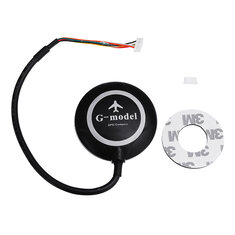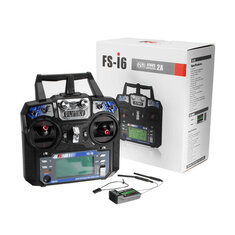………………………………………………………………………………………………………………………………………………………………………………………………………………………………………………………………………………………………………………….

#### WiringNext step is :

Select baud rate 57600Map column left to right with serial printMin :1050  Max : 1950 all channel.

Calibrate sensor.

Magneto

Turn Quad copter by hand find Magneto value

Xmin Xmax

Ymin,Ymax

Zmin,Zmax  lay down quad   +/- 90### NANO DRONE EP3 : Connect +BARO+GYRO+GPS

WiringCode

This code not professional code for flight controller just example for learn how to add all sensor please under stand !!

### NANO DRONE EP1 : Hook up Flysky to Arduino NANO##### Wiring##### Bind Remote ( Connect Transmitter and receiver )

```int channel1 = 2; //   Pitch
int channel2 = 3;//
int channel3 = 4; //  Throttle
int channel4 = 5;//    YAW
int SWA = 6;// SWA

int Channel1 = 0 ; // Used later to
int Channel2 = 0 ; // store values
int Channel3 = 0 ; // Used later to
int Channel4 = 0 ; // store values
int SWAstate = 0 ; // store values

void setup() {
// put your setup code here, to run once:
Serial.begin(9600);
pinMode (channel1, INPUT);// initialises the channels
pinMode (channel2, INPUT);// as inputs
pinMode (channel3, INPUT);// initialises the channels
pinMode (channel4, INPUT);// as inputs
pinMode (SWA,INPUT);// Switch A

}

void loop() {
// put your main code here, to run repeatedly:

Channel1 = (pulseIn (channel1, HIGH)); // Checks the value of channel1
Serial.print ("Channel1 :");
Serial.println (Channel1); //Prints the channels value on the serial monitor

Channel2 = (pulseIn (channel2, HIGH)); // Checks the value of channel1
Serial.print ("Channel2 :");
Serial.println (Channel2); //Prints the channels value value on the serial monitor

Channel3 = (pulseIn (channel3, HIGH)); // Checks the value of channel1
Serial.print ("Channel3 :");
Serial.println (Channel3); //Prints the channels value value on the serial monitor

Channel4 = (pulseIn (channel4, HIGH)); // Checks the value of channel1
Serial.print ("Channel4 :");
Serial.println (Channel4); //Prints the channels value value on the serial monitor
int SWAState = (pulseIn (SWA,HIGH)); // Checks the value of channel1
Serial.print ("SWAState :   ");
Serial.println (SWAState); //Prints the channels value value on the serial monitor

delay(2000);
}

```

### Quad copter Arduino NANO : Test and Calibration ESC

https://en.wikipedia.org/wiki/Aircraft_principal_axes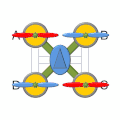Remote control FlySky   FS-I6C  TX , FS-IA6B RX#### Flysky FS-I6 TX   remote control manual

reference

http://www.electronoobs.com/eng_robotica_tut5_3.php

Test ESC and MOTOR and Direction```/*
Arduino Brushless Motor Control
by Dejan, https://howtomechatronics.com

and Modify by A-Arduino  :    www.a-arduino.com

*/
#include <Servo.h>
Servo ESC1;     // create servo object to control the 4 ESC
Servo ESC2;
Servo ESC3;
Servo ESC4;
int potValue;  // value from the analog pin
void setup() {
// Attach the ESC on pin 9
ESC1.attach(A1,1000,2000); // (pin A1, min pulse width, max pulse width in microseconds)
ESC2.attach(A2,1000,2000); // (pin A2, min pulse width, max pulse width in microseconds)
ESC3.attach(A3,1000,2000); // (pin A3, min pulse width, max pulse width in microseconds)
ESC4.attach(A4,1000,2000); // (pin A4, min pulse width, max pulse width in microseconds)
}
void loop() {
potValue = analogRead(A0);   // reads the value of the potentiometer (value between 0 and 1023)
potValue = map(potValue, 0, 1023, 0, 180);   // scale it to use it with the servo library (value between 0 and 180)
ESC1.write(potValue);    // Send the signal to the ESC
ESC2.write(potValue);    // Send the signal to the ESC
ESC3.write(potValue);    // Send the signal to the ESC
ESC4.write(potValue);    // Send the signal to the ESC
}```

### 3018 Mini CNC Upload GRBL program for control Mini CNCThe first thing for my friend to do before order mini cnc is this machine have no program include in this package you have to in stall GRBL to control

Procedure to include in Sketch  library

GRBL meaning

This table show example data when we install in the begining  this value machine can not run correctly have to adjust the important think that we need is the meaning of each parameter show in table below original information from

https://github.com/gnea/grbl/wiki/Grbl-v1.1-Configuration#—view-grbl-settings

Settings and sample values Description
\$0=10 Step pulse, microseconds
\$1=25 Step idle delay, milliseconds
\$4=0 Step enable invert, boolean
\$5=0 Limit pins invert, boolean
\$6=0 Probe pin invert, boolean
\$11=0.010 Junction deviation, mm
\$12=0.002 Arc tolerance, mm
\$13=0 Report inches, boolean
\$20=0 Soft limits, boolean
\$21=0 Hard limits, boolean
\$22=1 Homing cycle, boolean
\$24=25.000 Homing feed, mm/min
\$25=500.000 Homing seek, mm/min
\$26=250 Homing debounce, milliseconds
\$27=1.000 Homing pull-off, mm
\$30=1000. Max spindle speed, RPM
\$31=0. Min spindle speed, RPM
\$32=0 Laser mode, boolean
\$100=250.000 X steps/mm
\$101=250.000 Y steps/mm
\$102=250.000 Z steps/mm
\$110=500.000 X Max rate, mm/min
\$111=500.000 Y Max rate, mm/min
\$112=500.000 Z Max rate, mm/min
\$120=10.000 X Acceleration, mm/sec^2
\$121=10.000 Y Acceleration, mm/sec^2
\$122=10.000 Z Acceleration, mm/sec^2
\$130=200.000 X Max travel, mm
\$131=200.000 Y Max travel, mm
\$132=200.000 Z Max travel, mm

How to change GRBL parameter

Optimize GRBL set up for 3810 GRBL mini CNC

\$0=10
\$1=25
\$2=0
\$3=5
\$4=0
\$5=0
\$6=0
\$10=3
\$11=0.010
\$12=0.002
\$13=0
\$20=0
\$21=0
\$22=0
\$23=0
\$24=25.000
\$25=500.000
\$26=250
\$27=1.000
\$30=1000
\$31=0
\$32=0
\$100=800.000
\$101=800.000
\$102=800.000
\$110=2500.000
\$111=2000.000
\$112=1500.000
\$120=20.000
\$121=20.000
\$122=10.000
\$130=300.000
\$131=200.000
\$132=44.000